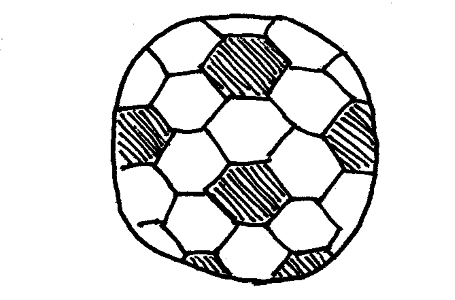# Football Soccer Arithmagon

Here are four new arithmagons for you to print on a Football Soccer themed worksheet. Use the buttons below the puzzles the shuffle the arithmagons to create four new puzzles, to view the solution, or to print as a worksheet.

## Football Soccer Arithmagons

Football, often called soccer is a sport played between eleven players where each team has to score a goal by getting the ball into the opposing goal.See if you can solve these four arithmagons.

The aim of an arithmagon is to work out which numbers go in the empty circles. The numbers in the square boxes are made by adding together the numbers in the circles either side. For example, if the number in the square box was 10 you could try 6 and 4, but the numbers must also add up on the other edges, making this more difficult than it first appears.